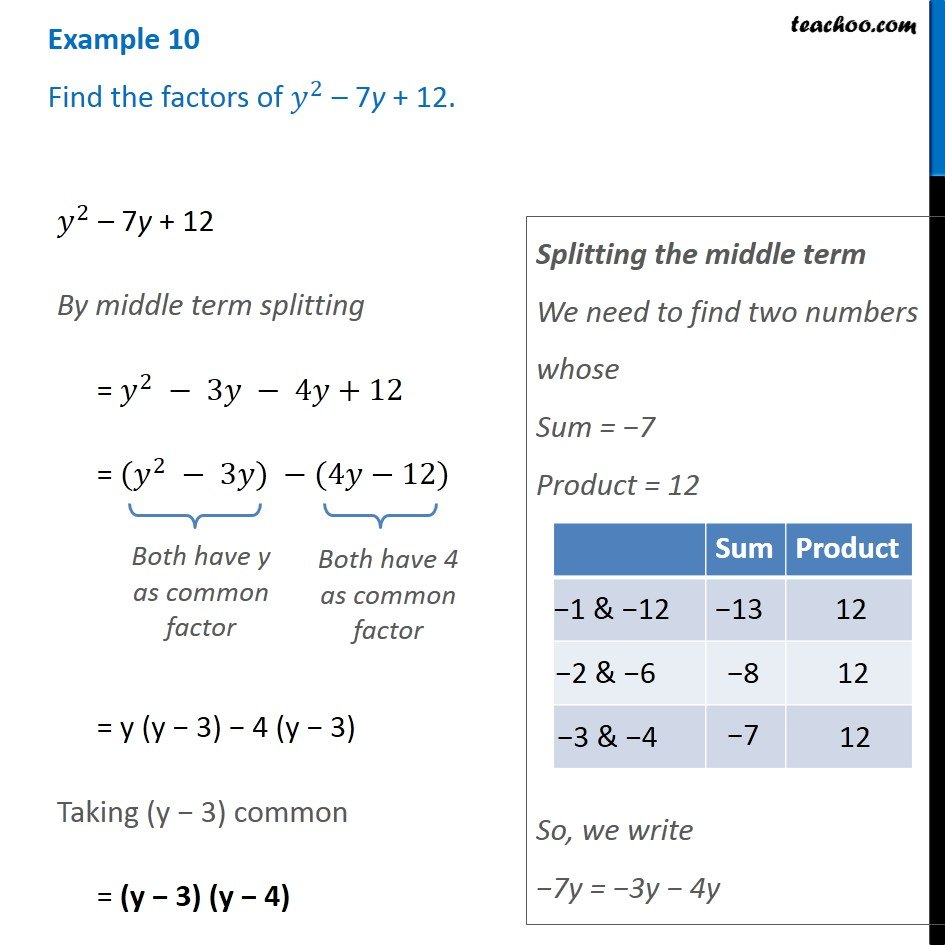Factorisation using splitting middle term

Chapter 14 Class 8 Factorisation
Concept wiseIntroducing your new favourite teacher - Teachoo Black, at only ₹83 per month

### Transcript

Example 10 Find the factors of 𝑦^2 – 7y + 12. 𝑦^2 – 7y + 12 By middle term splitting = 𝑦^2 − 3𝑦 − 4𝑦+12 = (𝑦^2 − 3𝑦) −(4𝑦−12) Both have y as common factor Both have 4 as common factor = y (y − 3) − 4 (y − 3) Taking (y − 3) common = (y − 3) (y − 4) Splitting the middle term We need to find two numbers whose Sum = −7 Product = 12 So, we write −7y = −3y − 4y